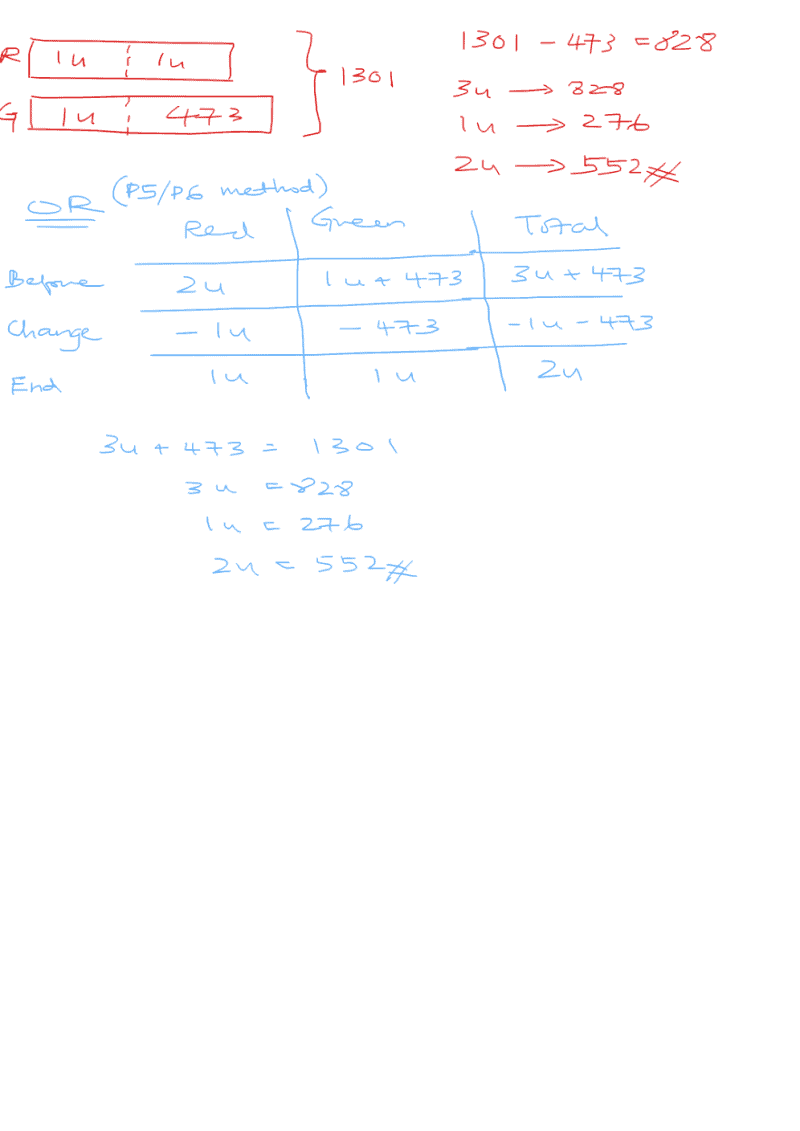# Question

Can someone help me with answer to this question?

Herman had 1301 red and green beads at first.he gave away half of his red beads and 473 of his green beads.in the end he had the same number of red and green beads left.how many red beads did he have at first

Hmm… I can easily solve this by algebra, but this is a P3 question, so I don’t think that is what you want.  But anyway, if R = Initial number of Red beads, and G = Initial number of Green beads, we will have

R + G = 1301

and 0.5R = G – 473

or G = 0.5R + 473

Substituting G into the first equation, we have:

R + 0.5R + 473 = 1301

or 1.5R = 1301 – 473 = 828

so R = 552

So he has 552 Red beads at first.

Can someone come up with a different solution for this, using the “recommended” way?